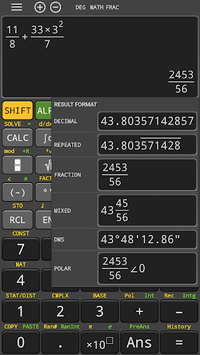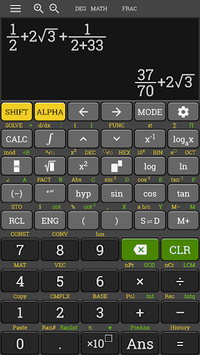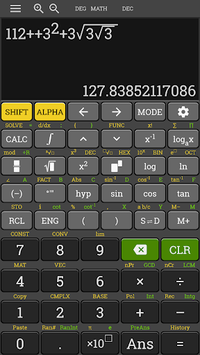# hp 35s fx Scientific Calculator 570 es plus free APK

The best calculator for Android full features of scientific calculator

### APK Details

 Category Education Last Updated 12-29-2018 Latest Version 3.8.1-beta-build-20-12-2018-17-release Size 12.77 MB Installations 50,000+ downloads Android version 4.0.3 and up Content Rating Everyone Ad Supported true Date Released Mar 1, 2018

### Screenshots## hp 35s fx Scientific Calculator 570 es plus free APK Description

Tavern Theme

The calculator retains the natural display, which means that you can enter equations and expressions exactly as written. Fractions, radicals, expressions with π, and calculus function templates are all there. Exact answers can include fractions, square roots, and coefficients of π. Supports most of the features of 82 500 570 991 4500 es ms, 580 vnx/ 580 vn.

All of the other features:
* Integrals of f(x)
* Numeric Derivatives
* Sums of a function
* Base modes Decimal, Octal, Binary, Hexadecimal
* Numeric solver, of equations and roots of expressions
* CALC button allows for calculating expressions repeated amount of times.
* Statistics including 1-variable, linear regression (a+bx), quadratic regression (a+bx+cx^2), cubic regression (a+bx+cx^2+dx^3), 2 types of exponential (a + b * e^x and a x^b), power (b a^x), logarithmic (a + b ln x), and inverse (a + b/x).
* Equations - 2x2 and 3x3 simultaneous equations, quadratic, and cubic equation
* Matrices: functions include transpose, inverse, and determinant
* Vectors
* Multi Line Statements with the colon (:)
* Complex Number Mode
* The number of available memories have increased from 7 to 9. (A, B, C, D, X, Y, M, and now E, F). Previously E and F were available only for the Hexadecimal mode.
* You now have the ability to calculate using repeated numbers. For example, you can type the decimal form of 1/3 using 0.3 with the bar above the three. I believe that this is first line of calculators that has this ability.
* New number functions are: GCD, LCM, Integer Part, Fractional Part, Random Integers, Integer Division (÷R) that gives quotient and remainder, and Prime Factorization (up to three digit factors). To factor a number, enter it, press [ = ], then [SHIFT], [ º ' '' ].
* Products of function f(x)
* In Table Mode you can include two functions f(x) and g(x).
* The rref and ref functions are added to the Matrix Mode (but not eigenvalues).
* The 115 es plus has a curve design, and boasts a faster processor.
* Inequality Solver of quadratic and cubic equations
* Verification mode, used for compare expressions (i.e. Does π/4 < π/2? Does 1 = 9/9 = e^0?)
* Distribution mode: Normal Distribution (CDF, PDF, and Inverse (Yes!)), Binomial Distribution (CDF, PDF), and Poisson Distribution (CDF, PDF).
* Support most of feature of hp 10 Scientific Calculator, hp 12C platinum financial calculator, hp 35 s scientific calculator, hp 17 financial calculator, hp 300 s Scientific Calculator, hp 12 C financial programmable calculator